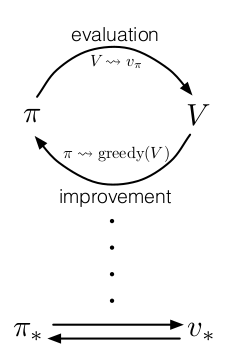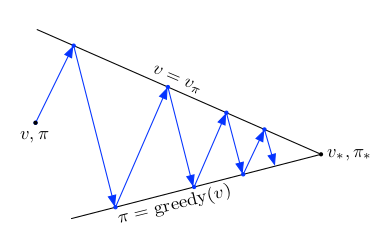[ 强化学习  ]

强化学习基础 3：Dynamic Programming

DP 的核心思想是利用价值函数来帮助最优策略的搜索（这也是强化学习中的核心思想之一）。本文将介绍如何使用 DP 来计算价值函数，并且进而获得最优策略。

一、策略评估（预测）

\begin{algorithm}
\caption{Iterative Policy Evaluation}
\begin{algorithmic}
\INPUT $\pi$, the policy to be evaluated\\
\INPUT $\theta>0$, a small threshold determining accuracy of estimation
\OUTPUT $V\approx v_\pi$

\PROCEDURE{PolicyEvaluation}{$\pi, \theta$}
\STATE Initialize $V(s)$, for all $s\in\mathcal{S}^+$, arbitrarily except that $V(terminal)=0$
\REPEAT
\STATE $\Delta \gets 0$
\FORALL{$s\in\mathcal{S}$}
\STATE $v\gets V(s)$
\STATE $V(s)\gets \sum_a\pi(a\mid s)\sum_{s',r}p(s',r\mid a, s)\Big[r+\gamma V(s')\Big]$
\STATE $\Delta\gets {\rm max}(\Delta, |v-V(s)|)$
\ENDFOR
\UNTIL{$\Delta<\theta$}
\RETURN $V$
\ENDPROCEDURE
\end{algorithmic}
\end{algorithm}

三、策略迭代

\begin{algorithm}
\caption{Policy Iteration}
\begin{algorithmic}
\OUTPUT $V\approx v_\ast$
\OUTPUT $\pi\approx\pi_\ast$
\STATE Initialize $\pi(s)\in\mathcal{A}(s)$, for all $s\in\mathcal{S}$
\REPEAT
\STATE $V\gets$ PolicyEvaluation($\pi,\theta$)
\STATE policy-stable $\gets$ \TRUE
\FORALL{$s\in\mathcal{S}$}
\STATE old-action $\gets\pi(s)$
\STATE $\pi(s)\gets \argmax_a\sum_{s',r}p(s',r\mid s,a)\left[r+V(s')\right]$
\IF{old-action $\ne\pi(s)$}
\STATE policy-stable $\gets$ \FALSE
\ENDIF
\ENDFOR
\UNTIL{policy-stable}
\RETURN $V,\pi$
\end{algorithmic}
\end{algorithm}

四、值迭代

\begin{algorithm}
\caption{Value Iteration}
\begin{algorithmic}
\INPUT $\theta>0$, a small threshold determining accuracy of estimation
\OUTPUT $\pi\approx \pi_\ast$

\STATE Initialize $V(s)$, for all $s\in\mathcal{S}^+$, arbitrarily except that $V(terminal)=0$
\REPEAT
\STATE $\Delta \gets 0$
\FORALL{$s\in\mathcal{S}$}
\STATE $v\gets V(s)$
\STATE $V(s)\gets \max_a\pi(a\mid s)\sum_{s',r}p(s',r\mid a, s)\Big[r+\gamma V(s')\Big]$
\STATE $\Delta\gets {\rm max}(\Delta, |v-V(s)|)$
\ENDFOR
\UNTIL{$\Delta<\theta$}
\RETURN $\pi(s)=\argmax_a\sum_{s',r}p(s',r\mid a, s)\Big[r+\gamma V(s')\Big]$
\end{algorithmic}
\end{algorithm}

六、GPI参考

• Richard S. Sutton. Reinforcement Learning: An Introduction